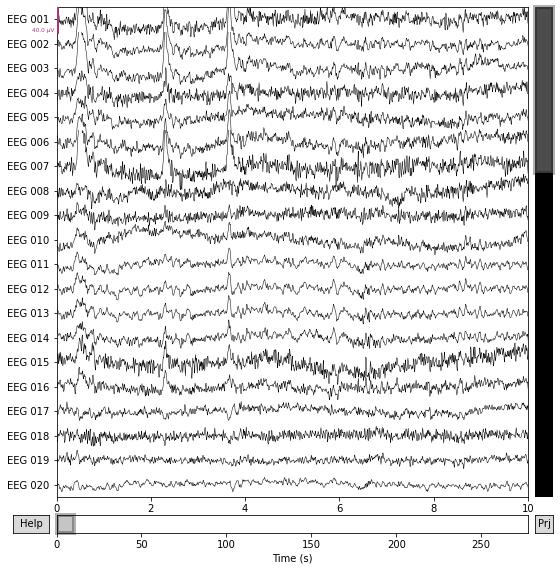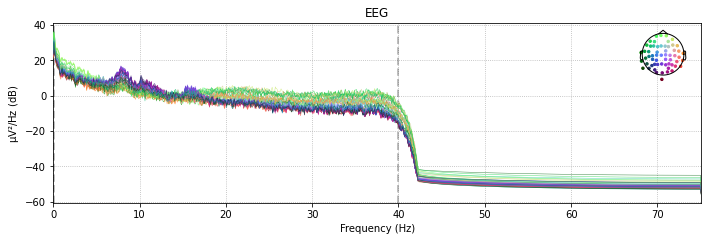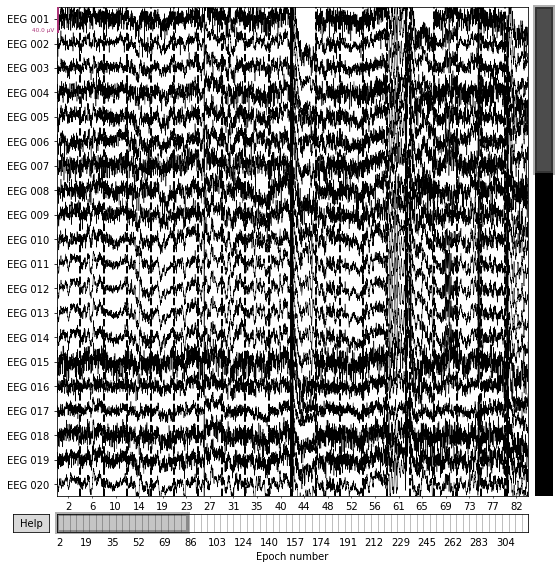×

Search anything:

# EEG Signal Analysis With Python

#### data science PythonGet this book -> Problems on Array: For Interviews and Competitive Programming

# Introduction

In this article, we will learn how to process EEG signals with Python using the MNE-Python library.

• Introduction to EEG
• Installation/Setup
• Plotting EEG Signals
• Preprocessing
• Epoching
• Conclusion

# Introduction to EEG

Electroencephalography (EEG) is a technique for continuously recording brain activity in the form of brainwaves. EEG is commonly used because it provides a noninvasive, easy, and inexpensive method to measure neural activity at a high resolution.

EEG analysis is used a lot in evaluating brain disorders, especially epilepsy or other seizure disorders. It is also used in brain-computer interfaces (BCIs).

EEG signals can be seen as a time series, since EEG recordings measure brain activity over a specific time period.

EEG devices are composed of different electrodes that are placed on the scalp. These electrodes are represented as channels using a montage. There are different types of montages, as described in detail in this video. A typical EEG system can have 1 to 256 channels. These channels are named based on their locations on the scalp.

# Installation/Setup

In this article, we will be using the MNE-Python library. It contains a lot of tools and algorithms we can use to easily analyze EEG/MEG recordings.

We can install MNE by using the following `pip` command:

``````pip install mne
``````

NumPy will also need to be installed:

``````pip install numpy
``````

### Import necessary modules/libraries:

``````import os
import numpy as np
import mne
``````

# Working with EEG Data

The MNE package supports various EEG file formats, including the following:

• European data format (.edf)
• EGI simple binary (.egi)
• EEGLAB set files (.set)

MNE has a sample dataset that we can use to become familiarized with processing EEG files. The below code shows how we can read a sample MEG/EEG file. There are different methods for different file formats. Since the sample file has the .fif extension, we call the `read_raw_fif` method. Also, since we are specifically focusing on EEG channels, we can exclude all non-EEG channels by using the `pick_types` method.

``````sample_data_folder = mne.datasets.sample.data_path()
sample_data_raw_file = os.path.join(sample_data_folder, 'MEG', 'sample', 'sample_audvis_filt-0-40_raw.fif')
raw = raw.pick_types(meg=False, eeg=True, eog=False, exclude='bads')
``````

This creates a `Raw` object (see here for more details).

We can inspect this `Raw` object by printing the `info` attribute (a dictionary-like object):

``````print(raw.info)
``````
``````Output:
<Info | 14 non-empty values
ch_names: EEG 001, EEG 002, EEG 003, EEG 004, EEG 005, EEG 006, EEG 007, ...
chs: 59 EEG
custom_ref_applied: False
dig: 146 items (3 Cardinal, 4 HPI, 61 EEG, 78 Extra)
file_id: 4 items (dict)
highpass: 0.1 Hz
hpi_meas: 1 item (list)
hpi_results: 1 item (list)
lowpass: 40.0 Hz
meas_date: 2002-12-03 19:01:10 UTC
meas_id: 4 items (dict)
nchan: 59
projs: Average EEG reference: off
sfreq: 150.2 Hz
>
``````

The `info` attribute keeps track of channel locations, recording date, number of channels, and more. Further detailed information on the `Info` structure can be found on MNE documentation here.

## Cropping Data

MNE `Raw` objects have a `crop` method that can be used to limit the data from the raw file to be between specific times (in seconds). This can help save memory.

``````raw.crop(0, 60) # keeps the first minute of data and discards the rest
``````

# Plotting EEG Signals

MNE has several methods to plot `Raw` objects. Here's one to generate a plot of the raw data:

``````raw.plot()
``````We can also plot the Power Spectral Density (PSD) for each channel. PSD shows the power as a function of frequency and is measured in power per unit frequency. It shows at which frequencies variations are strong as well as at which frequencies variations are weak.

``````raw.plot_psd()
``````In interactive Python sessions, `plot` functions create interactive plots. These allow for scrolling, scaling, annotations, and more.

# Preprocessing

## Resampling

EEG recordings have a high temporal resolution, so they are often recorded at high sampling rates (eg. 1000 Hz or higher). Although this makes the recordings very precise, it also consumes more memory. In cases where highly precise timing is not needed, downsampling the EEG signal can help save a lot of computation time.

`Raw` objects have a `resample` method that can be used to convert from one sample rate to another:

``````raw.resample(600) #resamples to a rate of 600 Hz
``````
``````Output:
Measurement date	December 03, 2002 19:01:10 GMT
Experimenter	Unknown
Participant	Unknown
Digitized points	0 points
Good channels	59 EEG
EOG channels	Not available
ECG channels	Not available
Sampling frequency	600.00 Hz
Highpass	1.00 Hz
Lowpass	40.00 Hz
Projections	Average EEG reference : off
Filenames	sample_audvis_filt-0-40_raw.fif
Duration	00:01:00 (HH:MM:SS)
``````

## Filtering

EEG data can have various artifacts and noise, so preprocessing must be done in order to maximize the signal-to-noise ratio (SNR), which measures the ratio of the signal power to the noise power.

Filtering is one of several techniques used for noise reduction/artifact removal.

`Raw` objects have a `filter` method that takes two arguments - `lfreq` represents the lower pass-band edge, and `hfreq` that represents the upper pass-band edge.

### High-pass filtering

High-pass filtering attenuates frequencies below a certain cutoff frequency. The rest of the signal remains unchanged.

The code below filters the signal attenuates the signal below 1 Hz and leaves the rest of the signal unchanged. Since `hfreq` is `None`, there is no upper pass-band edge, so the signal is high-passed.

``````raw.filter(1., None)
``````

### Low-Pass Filtering

Low-pass filtering is essentially the opposite of high-pass filtering. Instead of attenuating parts of the signal below a certain frequency, it attenuates parts of the signal above a certain frequency. It's called low-pass because it lets frequencies lower than a certain cutoff pass.

The code below attenuates the parts of the signal above 50 Hz and leaves the rest unchanged. Since `lfreq` is `None`, there is no lower pass-band edge, so the signal is low-passed.

``````raw.filter(None, 50.)
``````

### Notch Filter (Band Stop Filter)

The notch filter is a combination of both low-pass and high-pass filters. It can attenuate signals within a specific range of frequencies. The range of frequencies that a band-stop filter attenuates is called the stopband.

`Raw` objects have a `notch_filter` method that takes in a specific frequency or a list of frequencies to attenuate the signal at.

``````raw.notch_filter(60)
``````

This above example, attenuates the signal at 60 Hz.

Notch filters are often used when removing power-line noise, which occurs at a frequency of 50 or 60 Hz depending on the recording location. There may be peaks found at the harmonic frequencies (the integer multiples of the the power-line frequency, eg. 60, 120, 180, etc).

We can apply the notch filter to each of these frequencies by using the `numpy.arange` method. It turns an array of evenly spaced values between the `start` (inclusive) and `stop` (exlusive) parameters it takes. These evenly spaced values have a distance of `step` between each other, which is another parameter `numpy.arange` takes.

The below code will attenuate the parts of the signal with frequencies 60, 120, 180, and 240.

``````# the first 60 is start (inclusive), 241 is stop (exlusive), and 60 is step
raw.notch_filter(np.arange(60, 241, 60))
``````

# Epoching

Epochs are equal-length segments of data extracted from continuous EEG data. Usually, epochs are extracted around stimulus events or responses, but sometimes sequential or overlapping epochs are used.

MNE has an `Epochs` object used to represent epoched data. `Epochs` objects are used in other steps of EEG analysis, including feature extraction, which is used in machine learning.

In order to create epoched data, MNE-Python requires a `Raw` object as well as an array of events.

## Events

Events in MNE provide a mapping between specific times during an EEG/MEG recording and what happened at those times. Events are stored as a 2-dimensional NumPy array.

There are two ways to create events: reading from a file/`Raw` object or creating equal-sized events.

We will be using a different sample recording because the one we were originally using does not contain any events.

``````sample_data_folder = mne.datasets.sample.data_path()
sample_data_raw_file = os.path.join(sample_data_folder, 'MEG', 'sample', 'sample_audvis_raw.fif')
``````

The following code reads events from a `Raw` object:

``````events = mne.find_events(raw)
``````
``````Output:
320 events found
Event IDs: [ 1  2  3  4  5 32]
``````

### Creating equal-length events

Sometimes, there may not be any events included in the raw EEG recording. In such cases, an array of equally-spaced events can be generated. The code below creates second-long events for the first 10 seconds of EEG data:

``````events = mne.make_fixed_length_events(raw, start=0, stop=10, duration=1.)
``````

To create events that overlap by a certain duration, we can specify this duration using the `overlap` parameter in our call to the `make_fixed_length_events` method:

``````events = mne.make_fixed_length_events(raw, start=0, stop=10, duration=1., overlap=0.5)
``````

## Creating Epoched Data from Events

After loading/creating events, creating an `Epochs` object is fairly simple:

``````epochs = mne.Epochs(raw, events, preload=True).pick_types(eeg=True)
``````

`preload=True` loads all epochs from disk when creating the `Epochs` object.

## Selecting Epochs

Now that we have the `Epochs` object with event labels, we can select epochs using square brackets.

For example, we can plot the epochs where the event label was '1' (these event labels have an actual meaning, but they are not shown here for simplicity):

``````epochs['1'].plot()
``````

Output:# Conclusion

In this article, we learned about EEG signals, how they can be loaded, analyzed, preprocessed, and more. Understanding how to process EEG signals is very helpful for tasks that build on top of it - one important example of this is training a machine learning model to classify EEG segments.